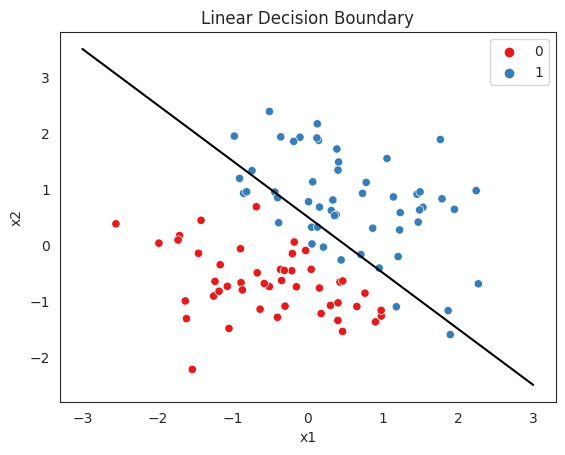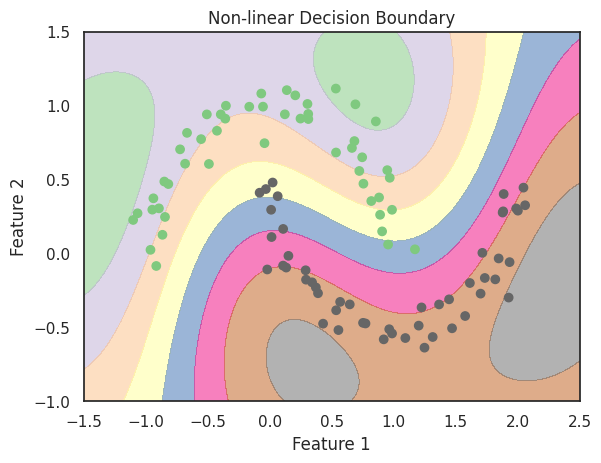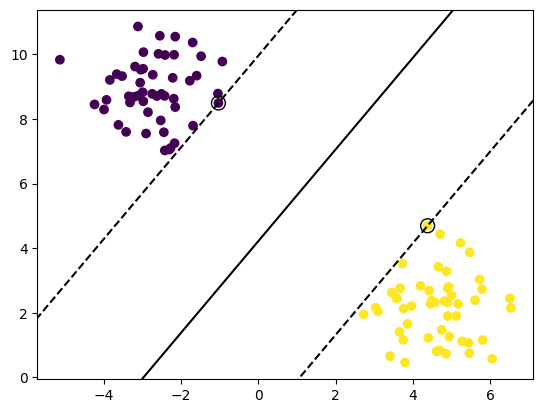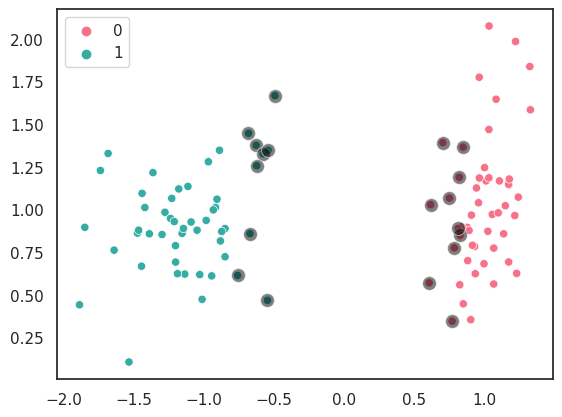×

Search anything:

# Decision Boundary in ML

#### Machine Learning (ML) Deep LearningIndian Technical Authorship Contest starts on 1st July 2023. Stay tuned.

Decision boundary is a crucial concept in machine learning and pattern recognition. It refers to the boundary or surface that separates different classes or categories in a classification problem. In simple terms, decision boundary is a line or curve that divides the data into two or more categories based on their features. The objective of decision boundary is to make accurate predictions on unseen data by identifying the correct class for a given input.

## What is Decision boundary

A hyperplane that partitions the feature space into distinct classes is known as a decision boundary. In binary classification problems, the decision boundary serves as the line of demarcation between positive and negative classes. The position and orientation of the decision boundary are determined by the model's training data and algorithm. The primary aim is to discover a decision boundary that can effectively generalize to new data, making it a reliable predictor.

## Types of Decision boundaries:

There are different types of decision boundaries based on the complexity of the classification problem. The most common types of decision boundaries are:

1. Linear decision boundary:
A linear decision boundary is a straight line that separates the data into two classes. It is the simplest form of decision boundary and is used when the classification problem is linearly separable. Linear decision boundary can be expressed in the form of a linear equation, y = mx + b, where m is the slope of the line and b is the y-intercept.2. Non Linear decision boundary:
A non-linear decision boundary is a curved line that separates the data into two or more classes. Non-linear decision boundaries are used when the classification problem is not linearly separable. Non-linear decision boundaries can take different forms such as parabolas, circles, ellipses, etc.3. Decision Boundary with Margin:
A decision boundary with margin is a line or curve that separates the data into two classes while maximizing the distance between the boundary and the closest data points. The margin is defined as the distance between the decision boundary and the closest data points of each class. The objective of decision boundary with margin is to improve the generalization performance of the classifier by reducing the risk of overfitting.4. Decision Boundary with Soft Margin:
A decision boundary with soft margin is a line or curve that separates the data into two classes while allowing some misclassifications. Soft margin is used when the data is not linearly separable and when the classification problem has some noise or outliers. The objective of decision boundary with soft margin is to find a balance between the accuracy of the classifier and its ability to generalize to unseen data.## How Decision Boundaries are Generated

There are different methods to generate decision boundaries depending on the type of classification problem and the algorithm used. The most common methods are:

1. Maximum Likelihood Estimation (MLE)
Maximum likelihood estimation is a statistical method used to estimate the parameters of a probability distribution based on the observed data. MLE is used to estimate the parameters of a model that generates the decision boundary that best fits the observed data.

2. Support Vector Machine (SVM)
Support vector machine is a supervised learning algorithm that is used to find the best decision boundary that separates the data into two or more classes. SVM uses a technique called kernel trick to transform the data into a higher-dimensional space where a linear decision boundary can be found.

3. Decision Trees
Decision trees are a type of supervised learning algorithm that is used to generate a decision boundary by recursively partitioning the feature space into smaller subspaces. The decision boundary is represented by a tree-like structure where each node represents a decision based on a feature value and each leaf represents a class label.

4. Neural Networks
Neural networks are a type of supervised learning algorithm that is used to generate decision boundaries by learning a non-linear mapping between the input features and the output classes. Neural networks consist of multiple layers of interconnected nodes that perform non-linear transformations on the input data to generate the output classes.

## Applications of Decision Boundaries

Decision boundaries are used in a wide range of applications in machine learning and pattern recognition. Some of the most common applications are:

1. Image Classification
Image classification is the process of assigning a label to an image based on its features. Decision boundaries are used to separate the different classes of images such as cats, dogs, flowers, etc. The decision boundary is learned from a training dataset of labeled images and is used to classify new unseen images.

2. Speech Recognition
Speech recognition is the process of transcribing spoken words into written text. Decision boundaries are used to identify the different phonemes and words in the speech signal. The decision boundary is learned from a training dataset of labeled speech signals and is used to recognize new unseen speech signals.

3. Fraud Detection
Fraud detection is the process of identifying fraudulent transactions in a financial system. Decision boundaries are used to distinguish between legitimate and fraudulent transactions based on their features such as amount, location, and time of the transaction. The decision boundary is learned from a training dataset of labeled transactions and is used to detect new unseen fraudulent transactions.

4. Medical Diagnosis
Medical diagnosis is the process of identifying the presence or absence of a disease based on the symptoms and medical tests. Decision boundaries are used to distinguish between different diseases based on their symptoms and test results. The decision boundary is learned from a training dataset of labeled medical cases and is used to diagnose new unseen medical cases.

## Challenges of Decision Boundaries

Although decision boundaries are a powerful tool in machine learning and pattern recognition, they face several challenges, including:

1. Overfitting
Overfitting occurs when the decision boundary is too complex and fits the training data too well, but fails to generalize to new unseen data. Overfitting can be addressed by using regularization techniques or by using simpler models.

2. Underfitting
Underfitting occurs when the decision boundary is too simple and fails to capture the underlying patterns in the data. Underfitting can be addressed by using more complex models or by adding more features to the dataset.

3. Class Imbalance
Class imbalance occurs when the dataset has an unequal distribution of samples across the different classes. Class imbalance can lead to biased decision boundaries that favor the majority class. Class imbalance can be addressed by using sampling techniques or by using different performance metrics.

## Summary

Decision boundary is a fundamental concept in machine learning and pattern recognition. It is used to separate the data into different classes based on their features. There are different types of decision boundaries, including linear, non-linear, decision boundary with margin, and decision boundary with soft margin. Decision boundaries are generated using different methods such as maximum likelihood estimation, support vector machine, decision trees, and neural networks. Decision boundaries are used in various applications such as image classification, speech recognition, fraud detection, and medical diagnosis. However, decision boundaries face several challenges such as overfitting, underfitting, and class imbalance. Addressing these challenges is crucial to build accurate and robust machine learning models.